RS Aggarwal Solutions: Coordinate Geometry- 3

# RS Aggarwal Solutions: Coordinate Geometry- 3 - RS Aggarwal Solutions for Class 10 Mathematics

## Exercise: 16d

Q.1. Points A(-1, y) and B(5, 7) lie on a circle with centre O(2, - 3y). Find the values of y.

The distance of any point which lies on the circumference of the circle from the centre of the circle is called radius.
∴ OA = OB = Radius of given Circle taking square on both sides, we get-
OA2 = OB2
⇒ (-1-2)2 + [y-(-3y)]2 = (5-2)2 + [7-(-3y)]2
[using distance formula, the distance between points (x1,y1) and (x2,y2) is equal to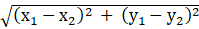units.]
⇒ 9 + 16y2 = 9 + (7 + 3y)2
⇒ 16y2 = 49 + 42y + 9y2
⇒ 7y2 - 42y - 49 = 0
⇒ 7(y2-6y-7) = 0
⇒ y2-7y + y-7 = 0
⇒ y(y-7) + 1(y-7) = 0
⇒ (y + 1)(y-7) = 0
∴ y = 7 or y = -1
Thus, possible values of y are 7 or -1.

Q.2. If the point A(0, 2) is equidistant from the points B(3, p) and C(p, 5), find p.

According to question-
AB = AC
taking square on both sides, we get-
AB2 = AC2
⇒ (0-3)2 + (2-p)2 = (0-p)2 + (2-5)2
[using distance formula, the distance between points (x1,y1) and (x2,y2) is equal tounits.]
⇒ 9 + 4 + p2 - 4p = p2 + 9
⇒ 4p-4 = 0
⇒ 4p = 4
∴ p = 1
Thus, the value of p is 1.

Q.3. ABCD is a rectangle whose three vertices are B(4, 0), C(4, 3) and D(0, 3). Find the length of one of its diagonal.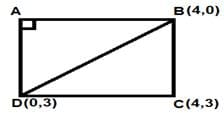fig.1
Clearly from fig.1, One of the diagonals of the rectangle ABCD is BD.
Length of diagonal BD is given by-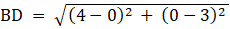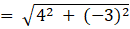=√(16 + 9)
= √25
= 5 units

Q.4. If the point P(k -1, 2) is equidistant from the points A(3, k) and B(k, 5), find the values of k.

According to question-
AP = BP
taking square on both sides, we get-
AP2 = BP2
⇒ (k-4)2 + (2-k)2 = (-1)2 + (2-5)2
[using distance formula, the distance between points (x1,y1) and (x2,y2) is equal tounits.]
⇒ k- 8k + 16 + 4 + k- 4k = 1 + 9
⇒ 2k2 - 12k + 20 = 10
⇒ 2k2 - 12k + 10 = 0
⇒ 2(k2-6k + 5) = 0
⇒ (k2-5k-k + 5) = 0
⇒ k(k-5)-1(k-5) = 0
⇒ (k-1)(k-5) = 0
∴ k = 1 or k = 5
Thus, the value of k is 1 or 5.

Q.5. Find the ratio in which the point P(x, 2) divides the join of A(12, 5) and B(4, -3).

Let the point P(x, 2) divides the join of A(12, 5) and B(4, -3) in the ratio of m:n.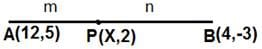fig.2
Recall that if (x, y) ≡ (a,b) then x = a and y = b
∴ assume that
(x, y) ≡ (x, 2)
(x1, y1) ≡ (12, 5)
and, (x2, y2) ≡ (4, -3)
Now, Using Section Formula-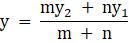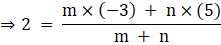⇒ 2m + 2n = -3m + 5n
⇒ 5m = 3n
∴ m:n = 3:5
Thus, the required ratio is 3:5.

Q.6. Prove that the diagonals of a rectangle ABCD with vertices A(2, -1), B(5, -1), C(5, 6) and D(2, 6) are equal and bisect each other.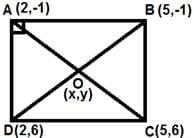fig.3
Length of diagonal AC is given by-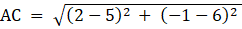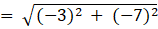=√(9 + 49)
= √58 units
Length of diagonal BD is given by-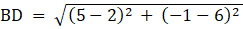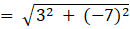= √(9 + 49)
= √58 units
Clearly, the length of the diagonals of the rectangle ABCD are equal.
Mid-point of Diagonal AC is given by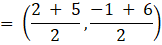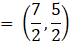Similarly, Mid-point of Diagonal BD is given by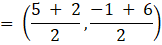Clearly, the coordinates of mid-point of both the diagonals coincide i.e. diagonals of the rectangle bisect each other.

Q.7. Find the lengths of the medians AD and BE of ΔABC whose vertices are A(7, -3), B(5, 3) and C(3, -1).

A median of a triangle is a line segment joining a vertex to the midpoint of the opposing side, bisecting it.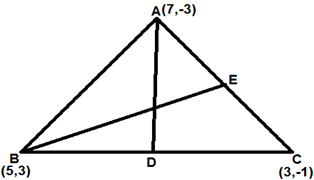fig.4
Mid-point of side BC opposite to vertex A i.e. coordinates of point D is given by-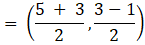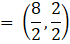= (4, 1)
Mid-point of side AC opposite to vertex B i.e. coordinates of point E is given by-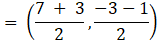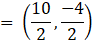= (5, -2)
Length of Median AD is given by-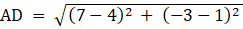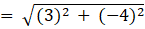= √(9 + 16)
= √25
= 5 units
Length of Median BE is given by-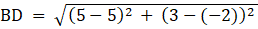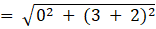= √(0 + 52 )
= √25
= 5 units
Thus, Length of Medians AD and BE are same which is equal to 5 units.

Q.8. If the point C(k, 4) divides the join of A(2, 6) and B(5, 1) in the ratio 2 : 3 then find the value of k.

Given that point C(k, 4) divides the join of A(2, 6) and B(5, 1) in the ratio 2 : 3.
∴ m:n = 2:3
Recall that if (x, y) ≡ (a, b) then x = a and y = b
Let (x, y) ≡ (k, 4)
(x1, y1) ≡ (2, 6)
and, (x2, y2) ≡ (5, 1)
Now, Using Section Formula-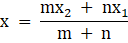On dividing numerator and denominator of R.H.S by n, we get-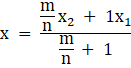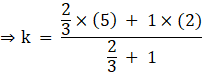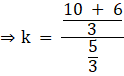∴ k = (16/5)
Thus the value of k is (16/5).

Q.9. Find the point on x-axis which is equidistant from points A(-1, 0) and B(5, 0).

Let the point on the x-axis which is equidistant from points A(-1,0) and B(5,0) i.e. the point which divides the line segment AB in the ratio 1:1 be C(x,0).
∴ m : n = 1:1
Recall that if (x,y) ≡ (a,b) then x = a and y = b
Let (x,y) ≡ (x,0)
(x1,y1) ≡ (-1,0)
and (x2,y2) ≡ (5,0)
Using Section Formula,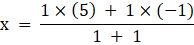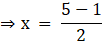⇒ x = (4/2) = 2
Thus, the point on the x-axis which is equidistant from points A(-1,0) and B(5,0) is P(2,0).

Q.10. Find the distance between the points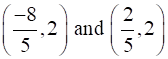.

The distance between the points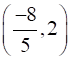and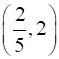is given by-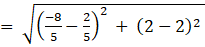[using distance formula, the distance between points (x1,y1) and (x2,y2) is equal tounits.]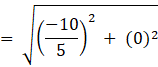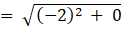=√4
= 2 units

Q.11. Find the value of a, so that the point (3, a) lies on the line represented by 2x - 3y = 5.

Since the point (3, a) lies on the line represented by 2x - 3y = 5
Thus, the point (3,a) will satisfy the above linear equation
∴ 2×(3) - 3×(a) = 5
⇒ 3a = 6-5
⇒ 3a = 1
∴ a = (1/3)
Thus, the value of a is (1/3).

Q.12. If the points A(4, 3) and B(x, 5) lie on the circle with centre 0(2, 3), find the value of x.

The distance of any point which lies on the circumference of the circle from the centre of the circle is called radius.
∴ OA = OB = Radius of given Circle taking square on both sides, we get-
OA2 = OB2
⇒ (2-4)2 + (3-3)2 = (2-x)2 + (3-5)2
[using distance formula, the distance between points (x1,y1) and (x2,y2) is equal tounits.]
⇒ (-2)2 + 0 = x2-4x + 4 + (-2)2
⇒ x2-4x + 4 = 0
⇒ (x-2)2 = 0
∴ x = 2
Thus, the value of x is 2.

Q.13. If P(x, y) is equidistant from the points A(7,1) and B(3, 5), find the relation between x and y.

According to question-
AP = BP
taking square on both sides, we get-
AP2 = BP2
⇒ (7-x)2 + (1-y)2 = (3-x)2 + (5-y)2
[using distance formula, the distance between points (x1,y1) and (x2,y2) is equal tounits.]
⇒ x2 - 14x + 49 + y2 - 2y + 1 = x2 - 6x + 9 + y2 - 10y + 25
⇒ -8x + 8y + 16 = 0
⇒ -8(x-y-2) = 0
⇒ x-y-2 = 0
∴ x-y = 2
This is the required relation between x and y.

Q.14. If the centroid of ΔABC having vertices A(a, b), B(b, c) and C(c, a) is the origin, then find the value of (a + b + c).

Every triangle has exactly three medians, one from each vertex, and they all intersect each other at a common point which is called centroid.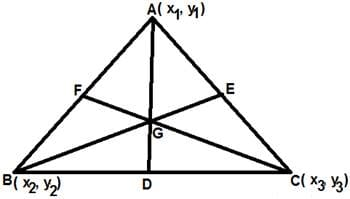fig.5
In the fig.5, Let AD, BE and CF be the medians of ΔABC and point G be the centroid.
We know that-
Centroid of a Δ divides the medians of the Δ in the ratio 2:1.
Mid-point of side BC i.e. coordinates of point D is given by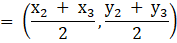Let the coordinates of the centroid G be (x,y).
Since centroid G divides the median AD in the ratio 2:1 i.e.
AG:GD = 2:1
∴ using section-formula, the coordinates of centroid is given by-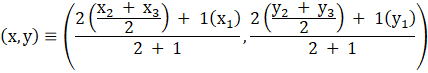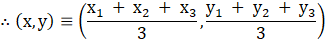Now, according to question-
Centroid of ΔABC having vertices A(a, b), B(b, c) and C(c, a) is the origin.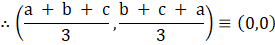Thus, the value of a + b + c is 0.

Q.15. Find the centroid of ΔABC whose vertices are A(2, 2), B(-4, -4) and C(5, - 8).

The centroid of a Δ whose vertices are (x1,y1), (x2,y2) and (x3,y3) is given by-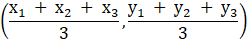∴ centroid of the given ΔABC ≡ [ (2-4 + 5)/3 , (2-4-8)/3 ]
≡ (1,-10/3)
Thus, the centroid of the given triangle ABC is (1,-10/3).

Q.16. In what ratio does the point C(4, 5) divide the join of A(2, 3) and B(7, 8)?

Let the ratio in which the point C(4, 5) divide the join of A(2, 3) and B(7, 8) be m:n.
Recall that if (x, y) ≡ (a, b) then x = a and y = b
Let (x, y) ≡ (4,5)
(x1, y1) ≡ (2,3)
and, (x2,y2) ≡ (7,8)
Now, Using Section Formula-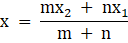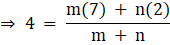⇒ 4m + 4n = 7m + 2n
⇒ 3m = 2n
∴ m:n = 2:3
Thus, the required ratio is 2:3.

Q.17. If the points A(2, 3), B(4, k) and C(6, -3) are collinear, find the value of k.

If the three points are collinear then the area of the triangle formed by them will be zero.
Area of a Δ ABC whose vertices are A(x1, y1), B(x2, y2) and C(x3, y3) is given by-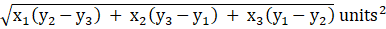∴ Area of given Δ ABC = 0
⇒ √(2(k-(-3)) + 4(-3-3) + 6(3-k) ) = 0
squaring both sides, we get-
2(k + 3) + 4(-6) + 6(3-k) = 0
⇒ 2k + 6-24 + 18-6k = 0
⇒ -4k + 24-24 = 0
∴ k = 0
Thus, the value of k is zero.

## Multiple Choice Questions (MCQ)

Question for RS Aggarwal Solutions: Coordinate Geometry- 3
Try yourself:The distance of the point P(-6, 8) from the origin is

Question for RS Aggarwal Solutions: Coordinate Geometry- 3
Try yourself:The distance of the point (-3, 4) from x-axis is

Question for RS Aggarwal Solutions: Coordinate Geometry- 3
Try yourself:The point on x-axis which is equidistant from points A(-1, 0) and B(5, 0) is

Question for RS Aggarwal Solutions: Coordinate Geometry- 3
Try yourself:If R(5, 6) is the midpoint of the line segment AB joining the points A(6, 5) and B(4, y) then y equals

Question for RS Aggarwal Solutions: Coordinate Geometry- 3
Try yourself:If the point C(k, 4) divides the join of the points A(2, 6) and B(5, 1) in the ratio 2 : 3 then the value of k is

Question for RS Aggarwal Solutions: Coordinate Geometry- 3
Try yourself:The perimeter of the triangle with vertices (0, 4), (0, 0) and (3, 0) is

Question for RS Aggarwal Solutions: Coordinate Geometry- 3
Try yourself:If A(1, 3), B(-1, 2), C(2, 5) and D(x, 4) are the vertices of a llgm ABCD then the value of x is

Question for RS Aggarwal Solutions: Coordinate Geometry- 3
Try yourself:If the points A(x, 2), B(-3, -4) and C(7, -5) are collinear then the value of x is

Question for RS Aggarwal Solutions: Coordinate Geometry- 3
Try yourself:The area of a triangle with vertices A(5, 0), B(8, 0) and C(8, 4) in square units is

Question for RS Aggarwal Solutions: Coordinate Geometry- 3
Try yourself:The area of ΔABC with vertices A(a, 0), O(0, 0) and B(0, b) in square units is

Question for RS Aggarwal Solutions: Coordinate Geometry- 3
Try yourself:If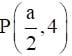is the midpoint of the line segment joining the points A(-6, 5) and B(-2, 3) then the value of a is

Question for RS Aggarwal Solutions: Coordinate Geometry- 3
Try yourself:ABCD is a rectangle whose three vertices are B(4, 0), C(4, 3) and D(0, 3). The length of one of its diagonals is

Question for RS Aggarwal Solutions: Coordinate Geometry- 3
Try yourself:The coordinates of the point P dividing the line segment joining the points A(1, 3) and B(4, 6) in the ratio 2: 1 is

Question for RS Aggarwal Solutions: Coordinate Geometry- 3
Try yourself:If the coordinates of one end of a diameter of a circle are (2, 3) and the coordinates of its center are (-2, 5), then the coordinates of the other end of the diameter are

Question for RS Aggarwal Solutions: Coordinate Geometry- 3
Try yourself:In the given figure P(5, -3) and Q(3, y) are the points of trisection of the line segment joining A(7, -2) and B(1, -5). Then, y equals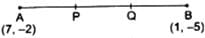Question for RS Aggarwal Solutions: Coordinate Geometry- 3
Try yourself:The midpoint of segment AB is P(0, 4). If the coordinates of B are (-2, 3), then the coordinates of A are

Question for RS Aggarwal Solutions: Coordinate Geometry- 3
Try yourself:The point P which divides the line segment joining the points A(2, -5) and B(5, 2) in the ratio 2 : 3 lies in the quadrant

Question for RS Aggarwal Solutions: Coordinate Geometry- 3
Try yourself:If A(-6, 7) and B(-1, -5) are two given points then the distance 2AB is

Question for RS Aggarwal Solutions: Coordinate Geometry- 3
Try yourself:Which point on the x-axis is equidistant from the points A(7, 6) and B(-3, 4)?

Question for RS Aggarwal Solutions: Coordinate Geometry- 3
Try yourself:The distance of P(3, 4) from the x-axis is

Question for RS Aggarwal Solutions: Coordinate Geometry- 3
Try yourself:In what ratio does the x-axis divide the join of A(2, -3) and B(5, 6)?

Question for RS Aggarwal Solutions: Coordinate Geometry- 3
Try yourself:In what ratio does the y-axis divide the join of P(-4, 2) and Q(8, 3)?

Question for RS Aggarwal Solutions: Coordinate Geometry- 3
Try yourself:If P(-1, 1) is the midpoint of the line segment joining A(-3, b) and B(1, b + 4) then b = ?

Question for RS Aggarwal Solutions: Coordinate Geometry- 3
Try yourself:The line 2x + y - 4 = 0 divides the line segment joining A(2, -2) and B(3, 7) in the ratio

Question for RS Aggarwal Solutions: Coordinate Geometry- 3
Try yourself:If A(4, 2), B(6, 5) and C(1, 4) be the vertices of ∆ABC and AD is a median, then the coordinates of D are

Question for RS Aggarwal Solutions: Coordinate Geometry- 3
Try yourself:If A(-1, 0), B(5, -2) and C(8, 2) are the vertices of a ∆ABC then its centroid is

Question for RS Aggarwal Solutions: Coordinate Geometry- 3
Try yourself:Two vertices of ΔABC are A (-1, 4) and B(5, 2) and its centroid is G(0, -3). Then, the coordinates of C are

Question for RS Aggarwal Solutions: Coordinate Geometry- 3
Try yourself:The points A(-4, 0), B(4, 0) and C(0, 3) are the vertices of a triangle, which is

Question for RS Aggarwal Solutions: Coordinate Geometry- 3
Try yourself:The points P(0, 6), Q(-5, 3) and R(3, 1) are the vertices of a triangle, which is

Question for RS Aggarwal Solutions: Coordinate Geometry- 3
Try yourself:If the points A(2, 3), B(5, k) and C(6, 7) are collinear then

Question for RS Aggarwal Solutions: Coordinate Geometry- 3
Try yourself:If the points A(1. 2), O(0, 0) and C(a, b) are collinear then

Question for RS Aggarwal Solutions: Coordinate Geometry- 3
Try yourself:The area of ΔABC with vertices A(3, 0), B(7, 0) and C(8, 4) is

Question for RS Aggarwal Solutions: Coordinate Geometry- 3
Try yourself:AOBC is a rectangle whose the vertices are A(0, 3), O(0, 0) and B(5, 0). The length of each of its diagonals is

Question for RS Aggarwal Solutions: Coordinate Geometry- 3
Try yourself:If the distance between the point A(4, p) and B(1, 0) is 5 then

The document RS Aggarwal Solutions: Coordinate Geometry- 3 | RS Aggarwal Solutions for Class 10 Mathematics is a part of the Class 10 Course RS Aggarwal Solutions for Class 10 Mathematics.
All you need of Class 10 at this link: Class 10

53 docs|15 tests

## RS Aggarwal Solutions for Class 10 Mathematics

53 docs|15 testsExplore Courses for Class 10 examSignup to see your scores go up within 7 days! Learn & Practice with 1000+ FREE Notes, Videos & Tests.
10M+ students study on EduRev
Track your progress, build streaks, highlight & save important lessons and more!
Related Searches

,

,

,

,

,

,

,

,

,

,

,

,

,

,

,

,

,

,

,

,

,

;